## RS Aggarwal Class 8 Solutions Chapter 1 Rational Numbers Ex 1A

These Solutions are part of RS Aggarwal Solutions Class 8. Here we have given RS Aggarwal Solutions Class 8 Chapter 1 Rational Numbers Ex 1A.

Other Exercises

Question 1.
Solution:
(i) 20 = 5 x 4
$$\\ \frac { -3 }{ 5 }$$ = $$\\ \frac { -3X4 }{ 5X4 }$$
(multiplying numerator and denominator by 4)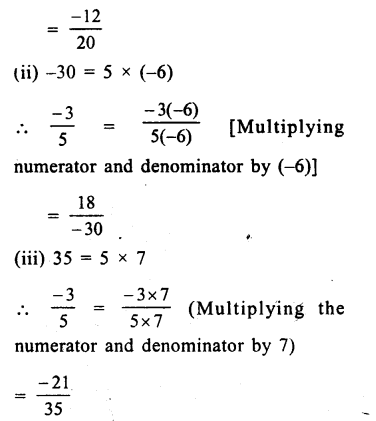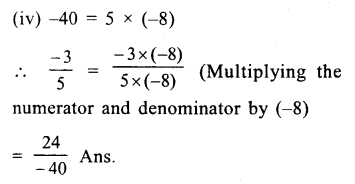Question 2.
Solution:
98 = 7 x 14Question 3.
Solution:
60 = 5 x 12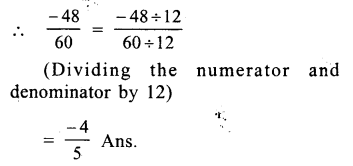Question 4.
Solution:
(i) $$\\ \frac { -12 }{ 30 }$$
H.C.F of 12 and 30 = 6
Dividing the numerator and denominator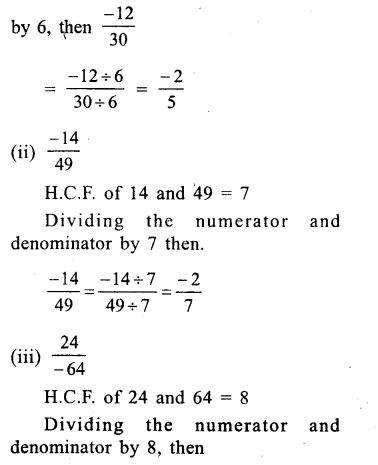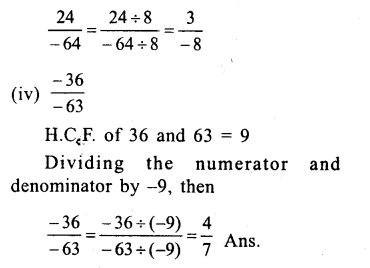Question 5.
Solution:
(i) $$\\ \frac { 3 }{ 8 }$$ or 0
We know that every positive rational number is greater than 0.
∴$$\\ \frac { 3 }{ 8 }$$ is greater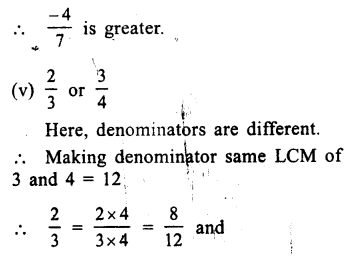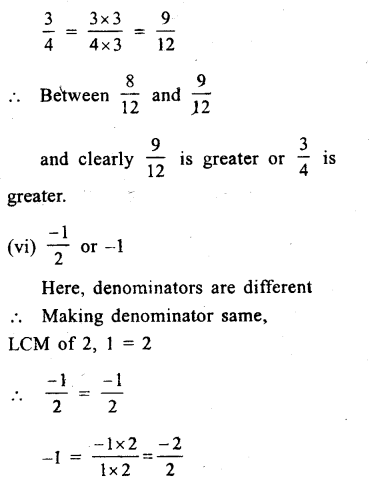and clearly $$\frac{-1}{2}$$ is greater. Ans.

Question 6.
Solution:
(i) $$\\ \frac { -4 }{ 3 }$$ or $$\\ \frac { 8 }{ 7 }$$
Her,denominator are not same
LCM of 3 and 7 = 21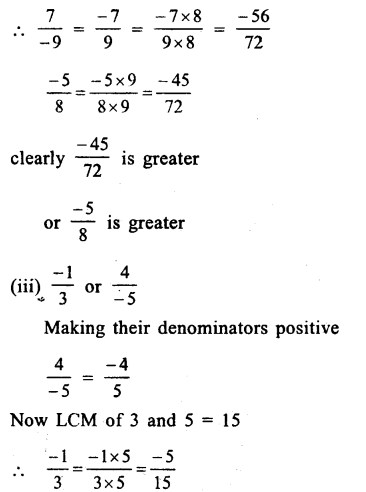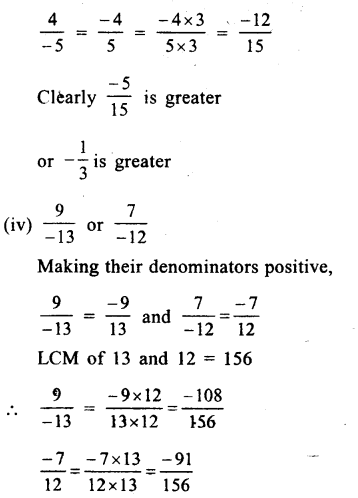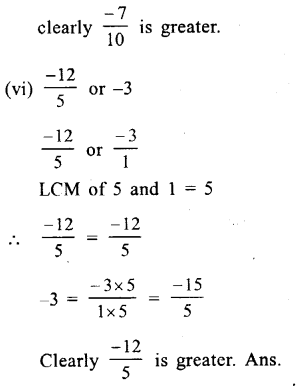Question 7.
Solution:
(i) Between $$\\ \frac { -3 }{ 7 }$$ and $$\\ \frac { 6 }{ -13 }$$
or $$\\ \frac { -3 }{ 7 }$$ and $$\\ \frac { -6 }{ 13 }$$
LCM of 7 and 13 = 91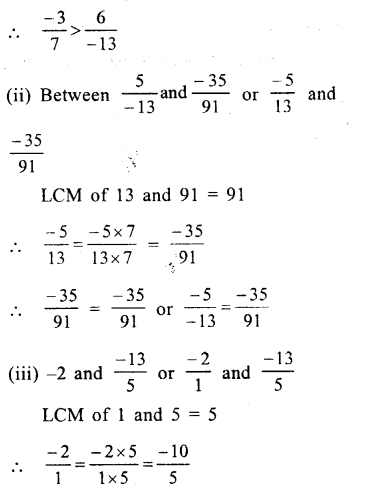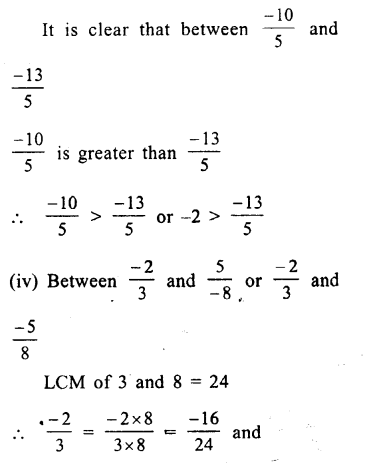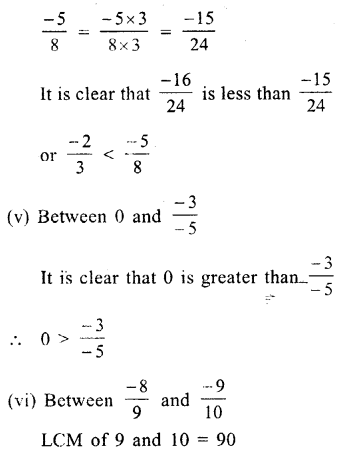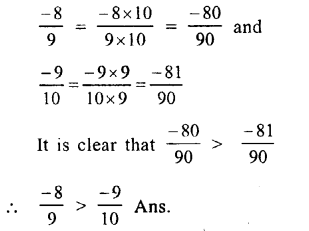Question 8.
Solution:
Among, $$\\ \frac { 4 }{ -9 }$$,$$\\ \frac { -5 }{ 12 }$$,$$\\ \frac { 7 }{ -18 }$$,$$\\ \frac { -2 }{ 3 }$$ making their denominator positive,we get: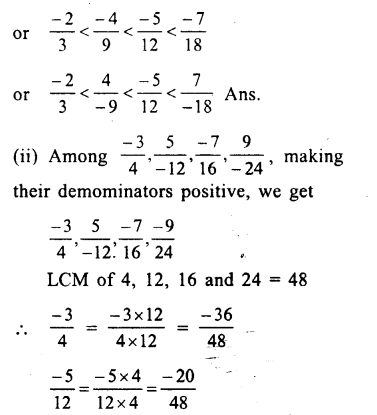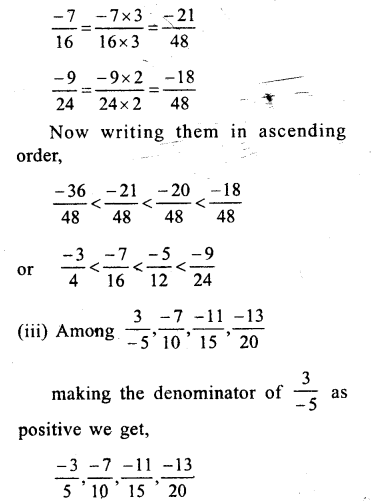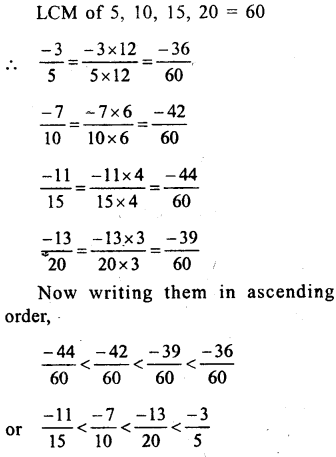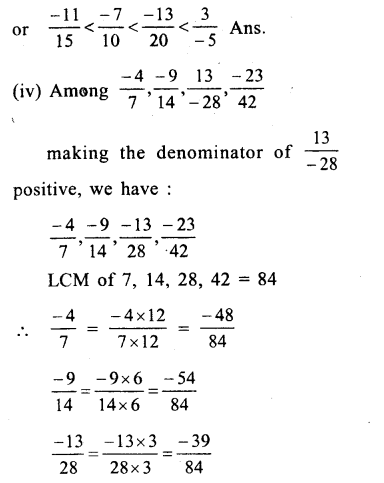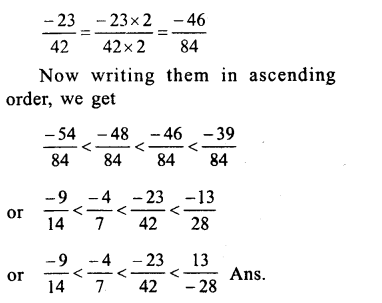Question 9.
Solution:
(i)Among – 2,$$\\ \frac { -13 }{ 6 }$$,$$\\ \frac { 8 }{ -3 }$$,$$\\ \frac { 1 }{ 3 }$$
Making the denominator of $$\\ \frac { 8 }{ -3 }$$ as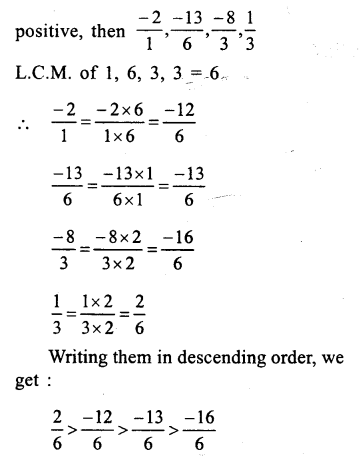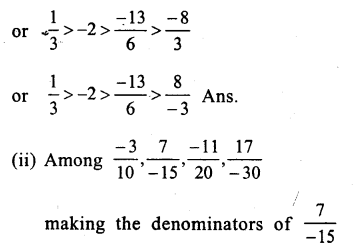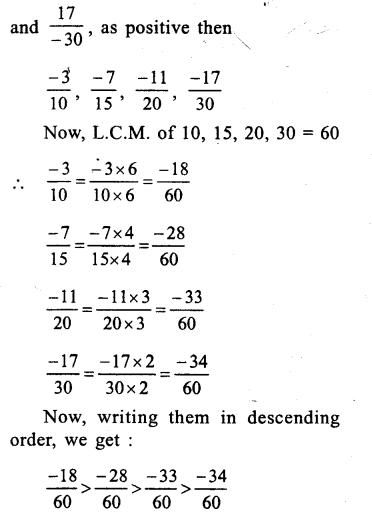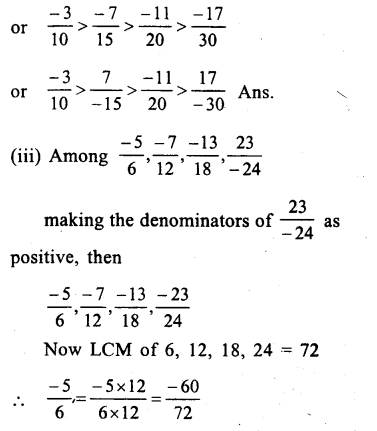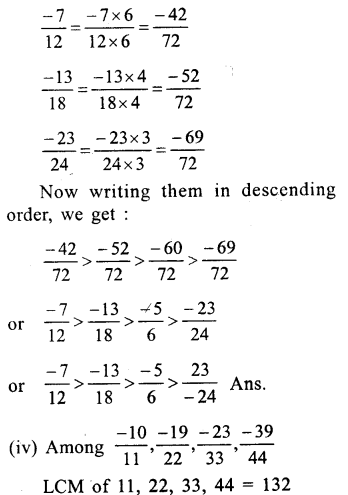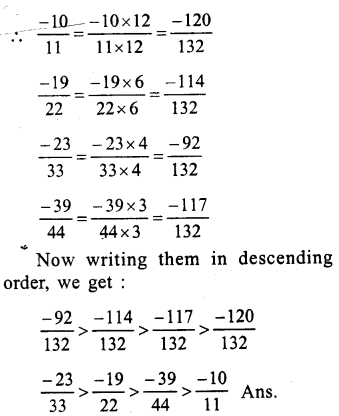Question 10.
Solution:
(i) True, as the set of whole numbers is a subset of the set of rational numbers
(ii) True, as the set of integers is a subset of the set of rational numbers.
(iii) False, as 0 is a whole number and set of the whole number is a subset of rational numbers
∴ 0 is also a rational number.

Hope given RS Aggarwal Solutions Class 8 Chapter 1 Rational Numbers Ex 1A are helpful to complete your math homework.

If you have any doubts, please comment below. Learn Insta try to provide online math tutoring for you.